Скачать презентацию Heat in Reactions Thermochemistry n The study

391479f2507bd7a8b785c1a85983f095.ppt

• Количество слайдов: 38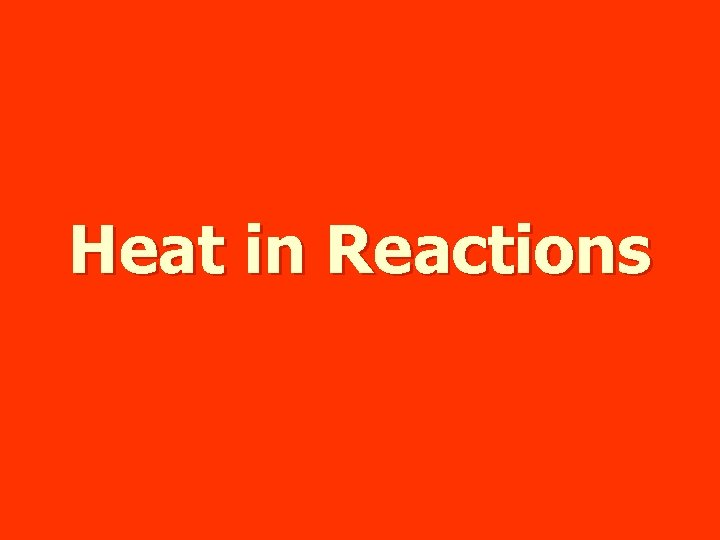Heat in Reactions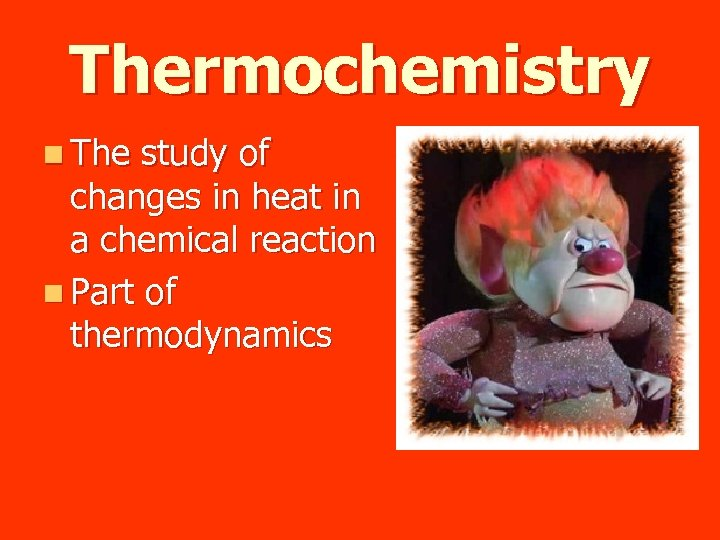Thermochemistry n The study of changes in heat in a chemical reaction n Part of thermodynamics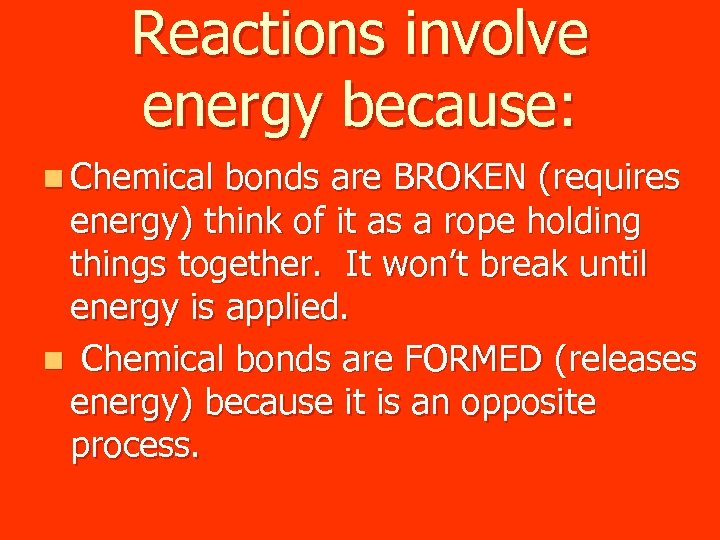Reactions involve energy because: n Chemical bonds are BROKEN (requires energy) think of it as a rope holding things together. It won’t break until energy is applied. n Chemical bonds are FORMED (releases energy) because it is an opposite process.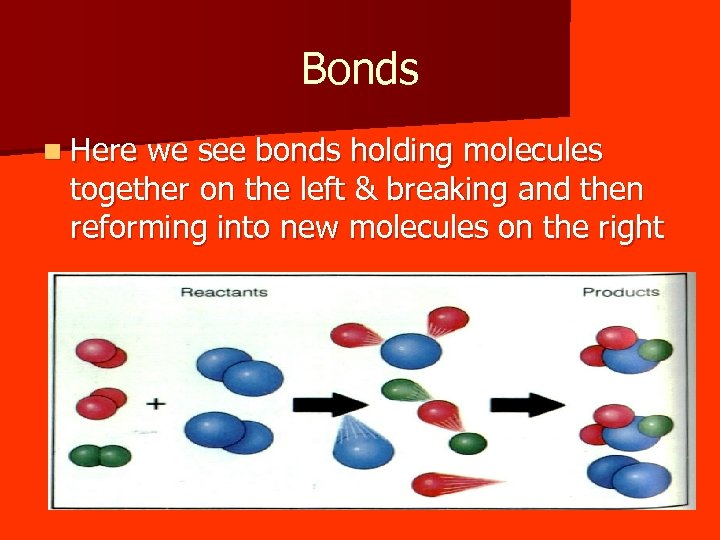Bonds n Here we see bonds holding molecules together on the left & breaking and then reforming into new molecules on the right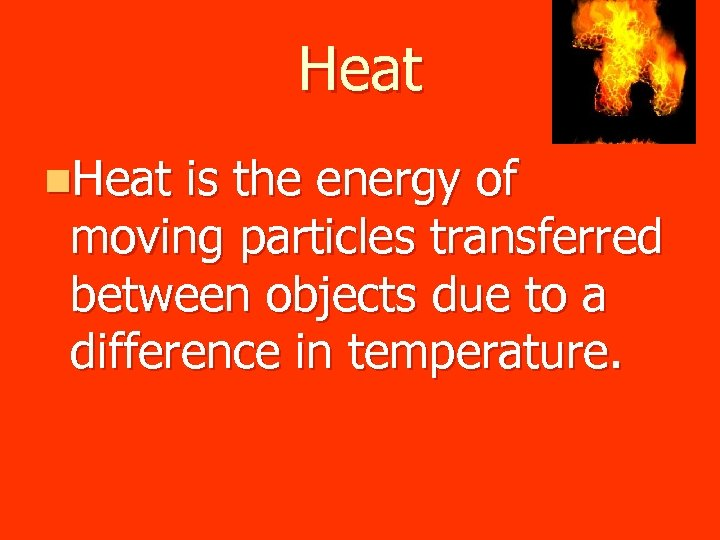Heat n. Heat is the energy of moving particles transferred between objects due to a difference in temperature.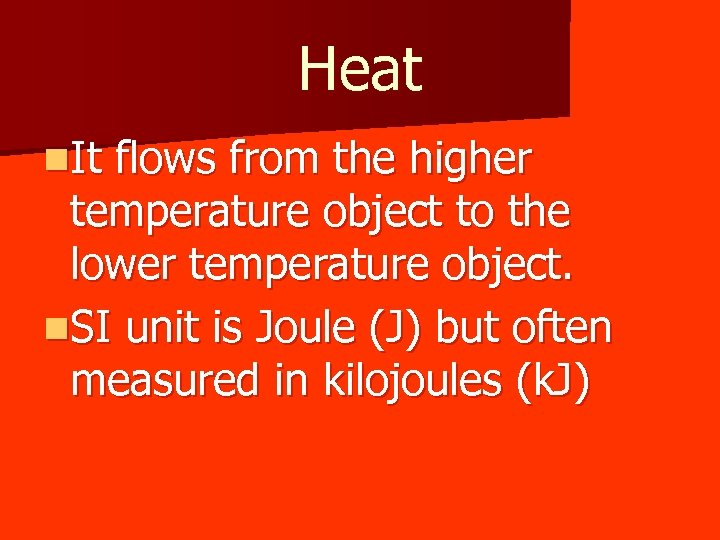Heat n. It flows from the higher temperature object to the lower temperature object. n. SI unit is Joule (J) but often measured in kilojoules (k. J)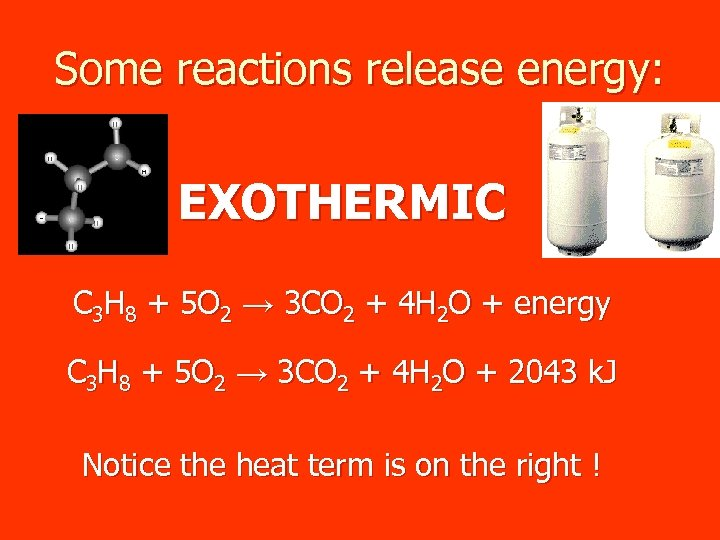Some reactions release energy: EXOTHERMIC C 3 H 8 + 5 O 2 → 3 CO 2 + 4 H 2 O + energy C 3 H 8 + 5 O 2 → 3 CO 2 + 4 H 2 O + 2043 k. J Notice the heat term is on the right !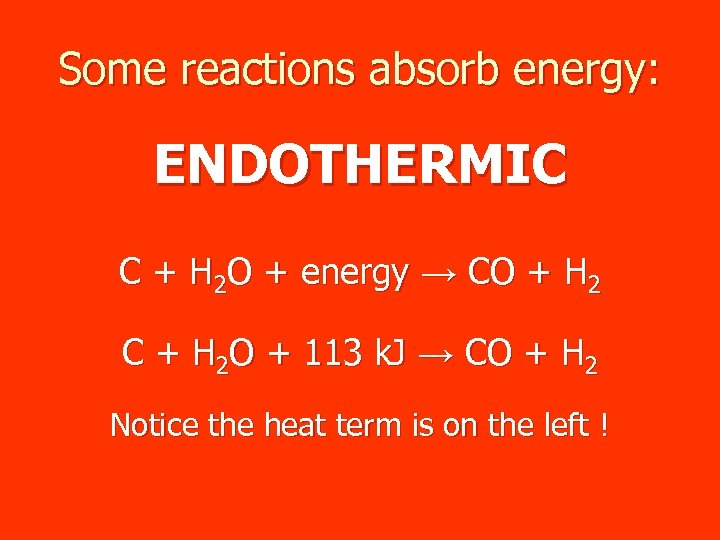Some reactions absorb energy: ENDOTHERMIC C + H 2 O + energy → CO + H 2 C + H 2 O + 113 k. J → CO + H 2 Notice the heat term is on the left !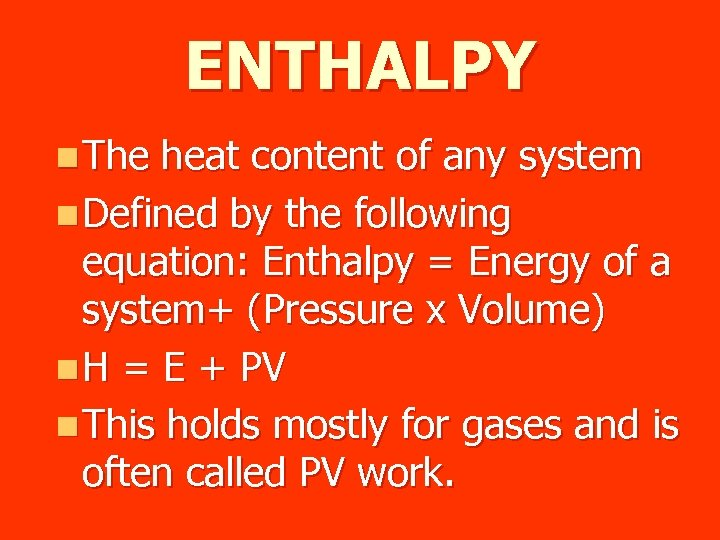ENTHALPY n The heat content of any system n Defined by the following equation: Enthalpy = Energy of a system+ (Pressure x Volume) n H = E + PV n This holds mostly for gases and is often called PV work.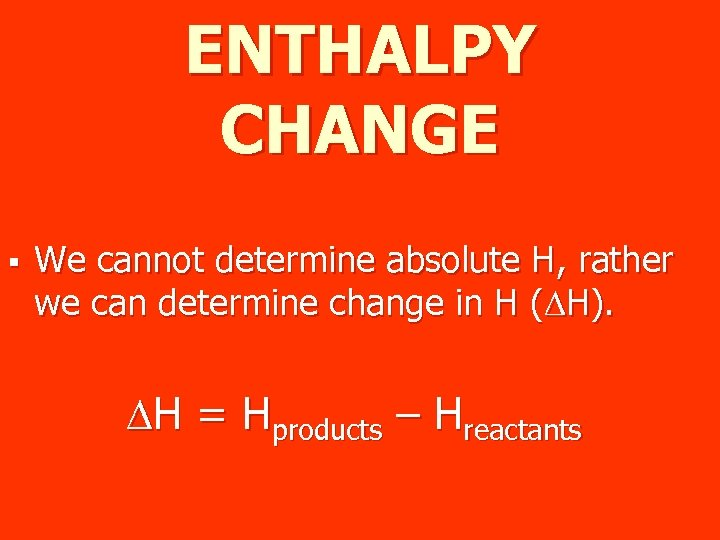ENTHALPY CHANGE § We cannot determine absolute H, rather we can determine change in H (DH). DH = Hproducts – Hreactants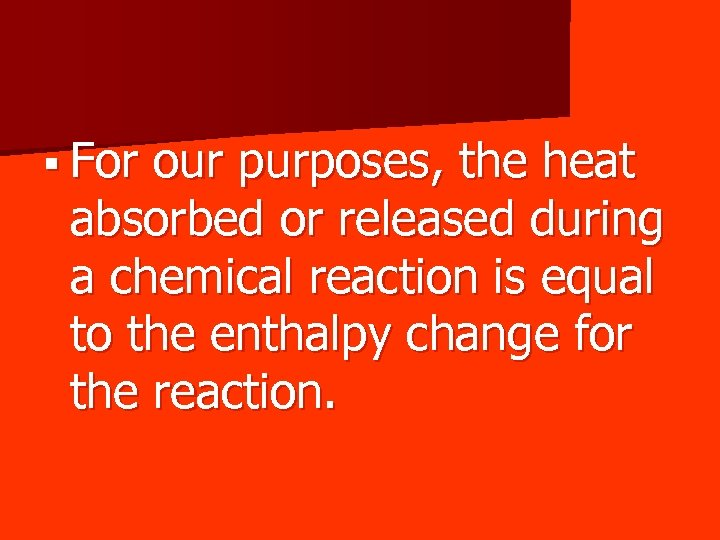§ For our purposes, the heat absorbed or released during a chemical reaction is equal to the enthalpy change for the reaction.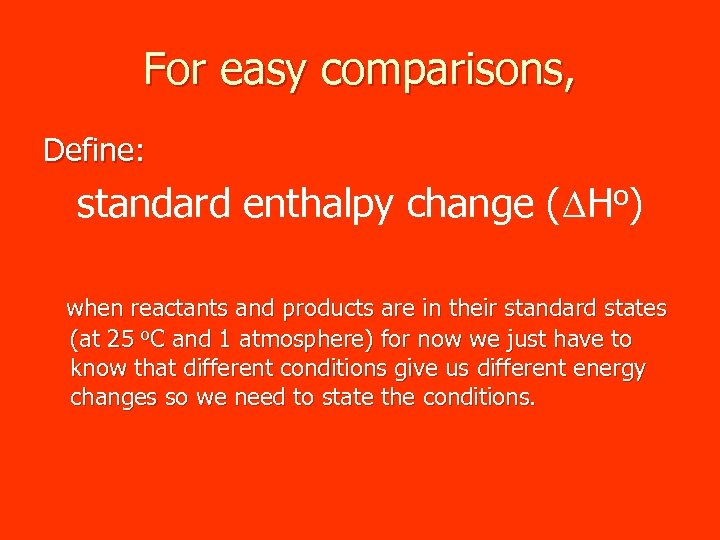For easy comparisons, Define: standard enthalpy change (DHo) when reactants and products are in their standard states (at 25 o. C and 1 atmosphere) for now we just have to know that different conditions give us different energy changes so we need to state the conditions.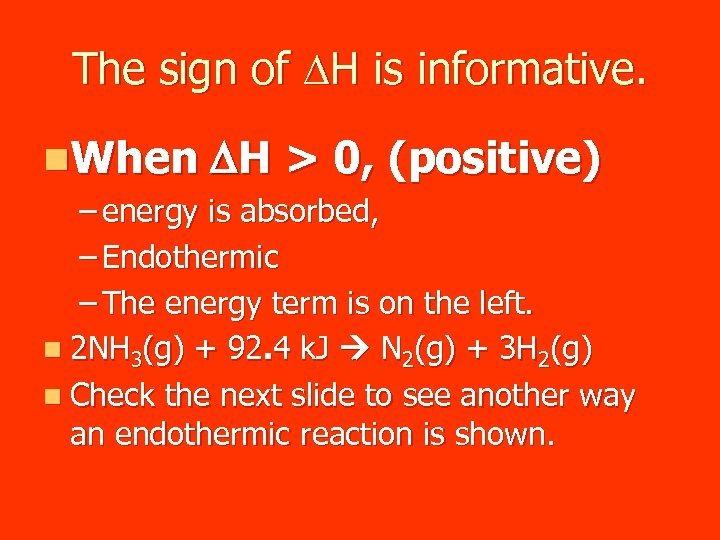The sign of DH is informative. n. When DH > 0, (positive) – energy is absorbed, – Endothermic – The energy term is on the left. n 2 NH 3(g) + 92. 4 k. J N 2(g) + 3 H 2(g) n Check the next slide to see another way an endothermic reaction is shown.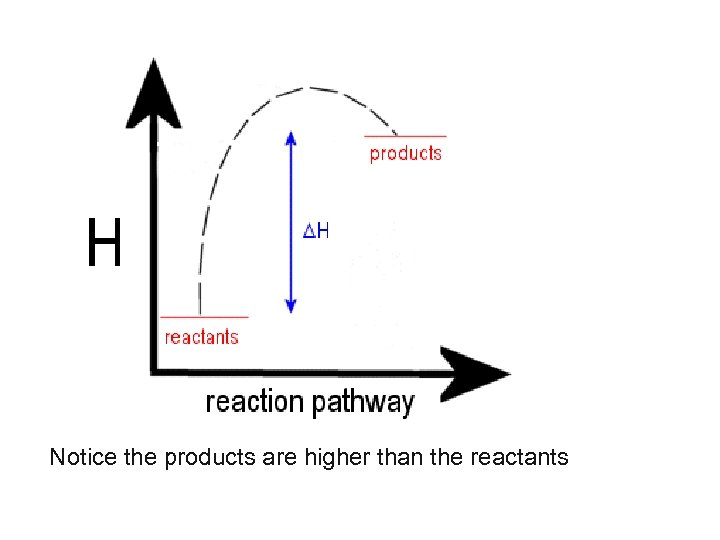Notice the products are higher than the reactants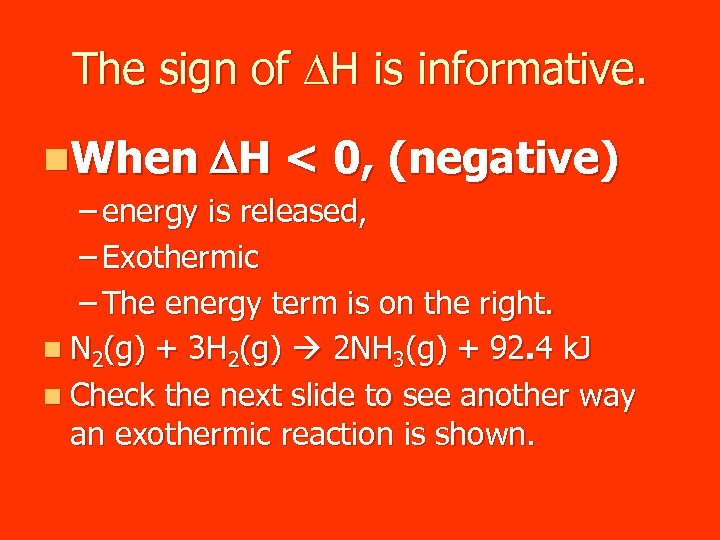The sign of DH is informative. n. When DH < 0, (negative) – energy is released, – Exothermic – The energy term is on the right. n N 2(g) + 3 H 2(g) 2 NH 3(g) + 92. 4 k. J n Check the next slide to see another way an exothermic reaction is shown.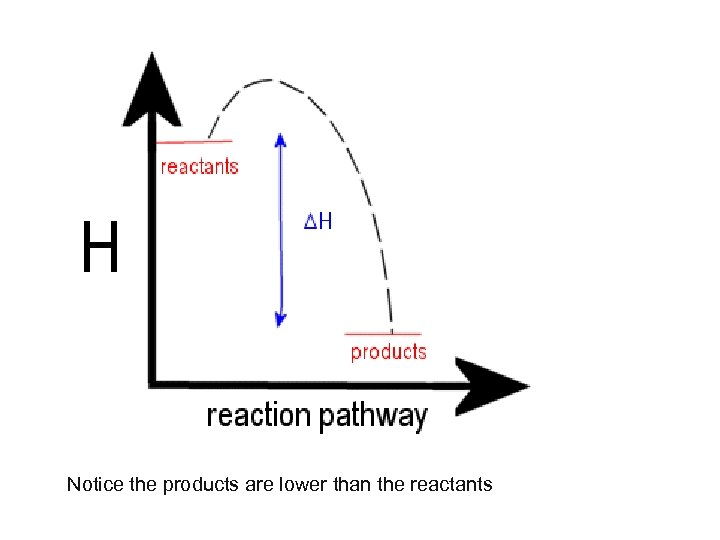Notice the products are lower than the reactants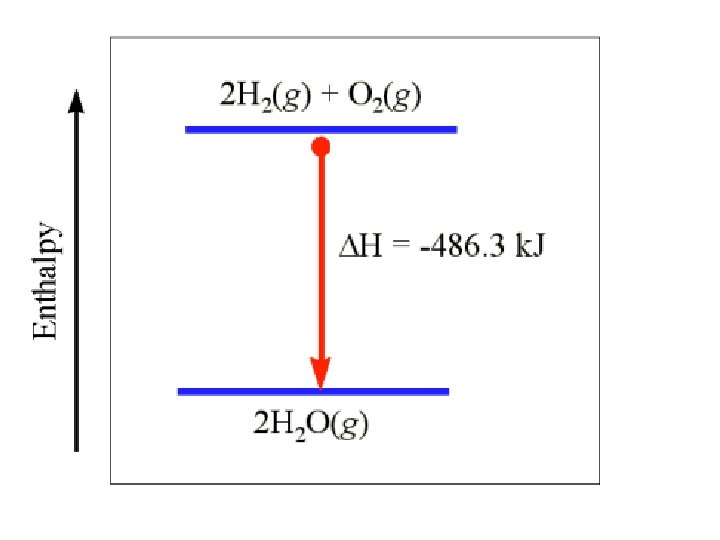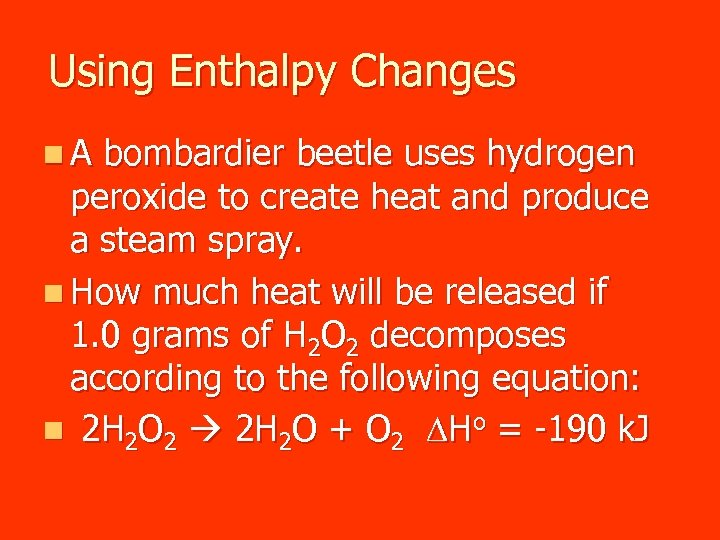Using Enthalpy Changes n. A bombardier beetle uses hydrogen peroxide to create heat and produce a steam spray. n How much heat will be released if 1. 0 grams of H 2 O 2 decomposes according to the following equation: n 2 H 2 O 2 2 H 2 O + O 2 DHo = -190 k. J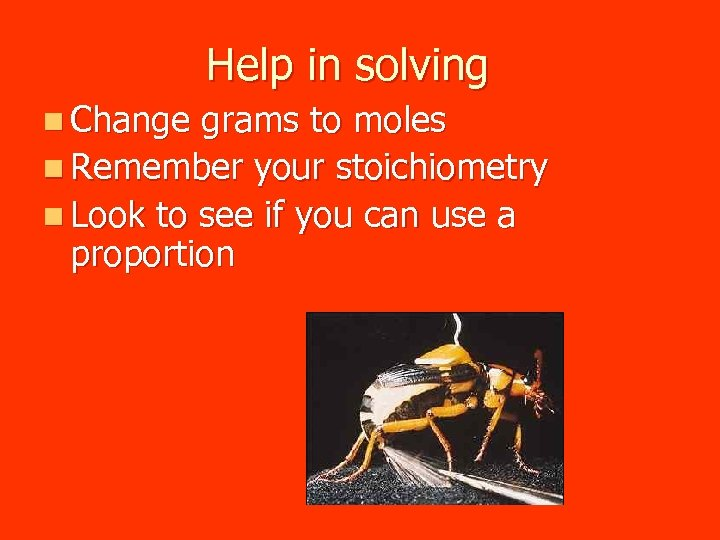Help in solving n Change grams to moles n Remember your stoichiometry n Look to see if you can use a proportion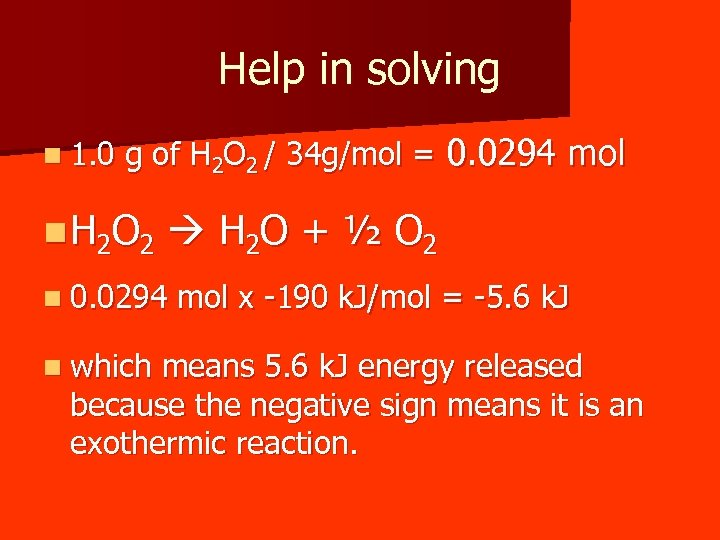Help in solving n 1. 0 g of H 2 O 2 / 34 g/mol = 0. 0294 mol n H 2 O 2 H 2 O + ½ O 2 n 0. 0294 n which mol x -190 k. J/mol = -5. 6 k. J means 5. 6 k. J energy released because the negative sign means it is an exothermic reaction.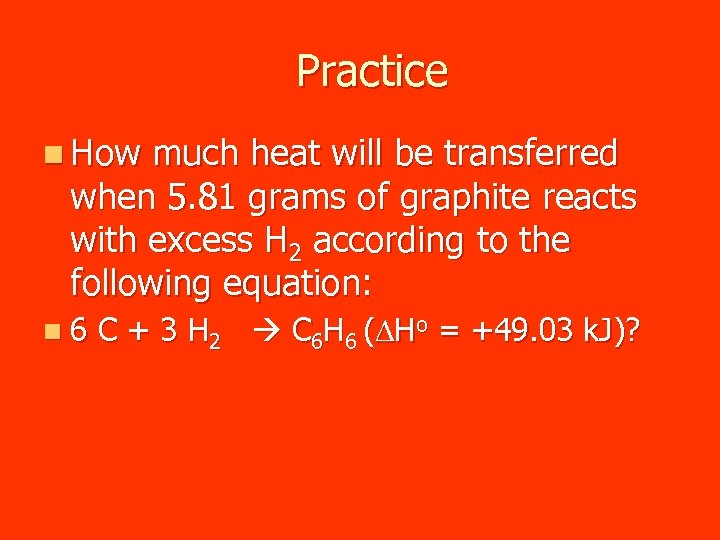Practice n How much heat will be transferred when 5. 81 grams of graphite reacts with excess H 2 according to the following equation: n 6 C + 3 H 2 C 6 H 6 (DHo = +49. 03 k. J)?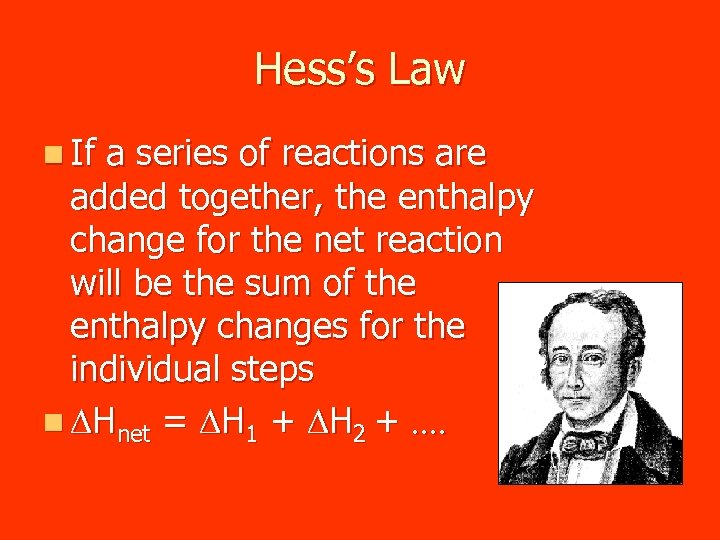Hess’s Law n If a series of reactions are added together, the enthalpy change for the net reaction will be the sum of the enthalpy changes for the individual steps n DHnet = DH 1 + DH 2 +. .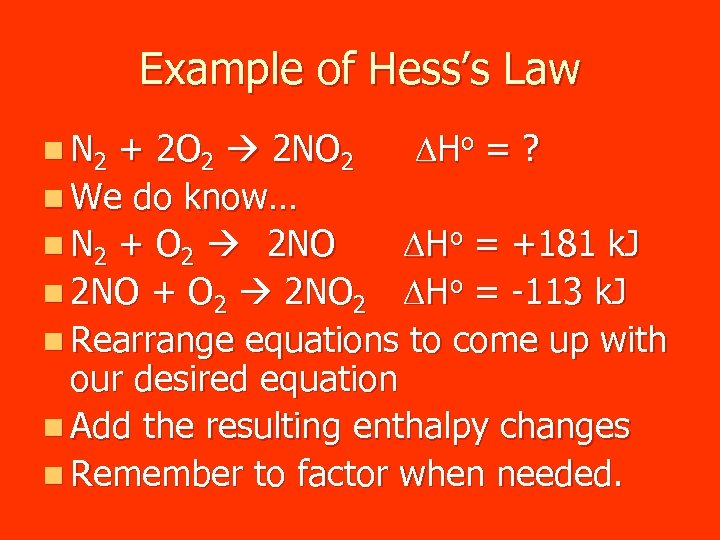Example of Hess’s Law + 2 O 2 2 NO 2 D Ho = ? n We do know… n N 2 + O 2 2 NO DHo = +181 k. J n 2 NO + O 2 2 NO 2 DHo = -113 k. J n Rearrange equations to come up with our desired equation n Add the resulting enthalpy changes n Remember to factor when needed. n N 2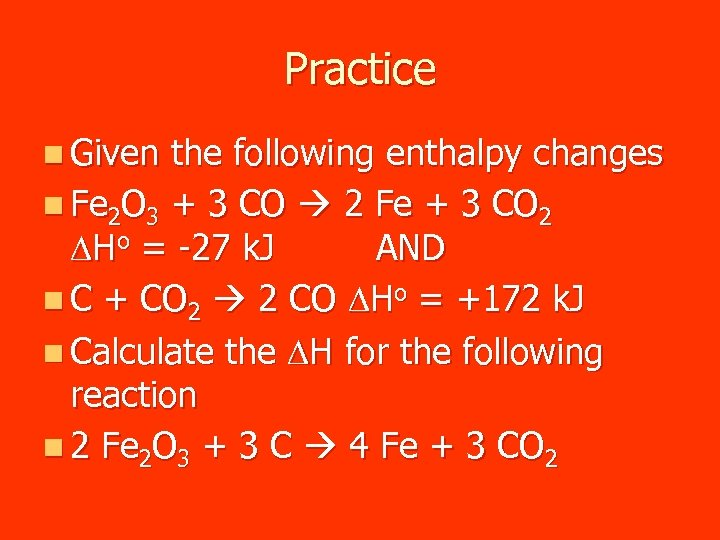Practice n Given the following enthalpy changes n Fe 2 O 3 + 3 CO 2 Fe + 3 CO 2 DHo = -27 k. J AND n C + CO 2 2 CO DHo = +172 k. J n Calculate the DH for the following reaction n 2 Fe 2 O 3 + 3 C 4 Fe + 3 CO 2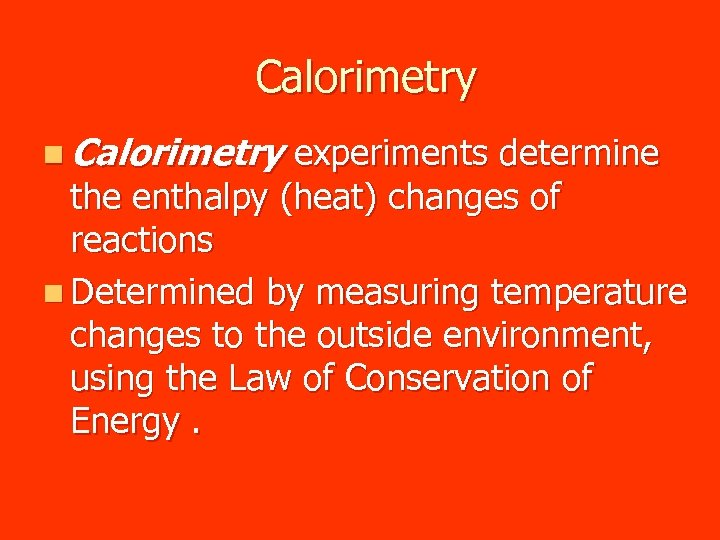Calorimetry n Calorimetry experiments determine the enthalpy (heat) changes of reactions n Determined by measuring temperature changes to the outside environment, using the Law of Conservation of Energy.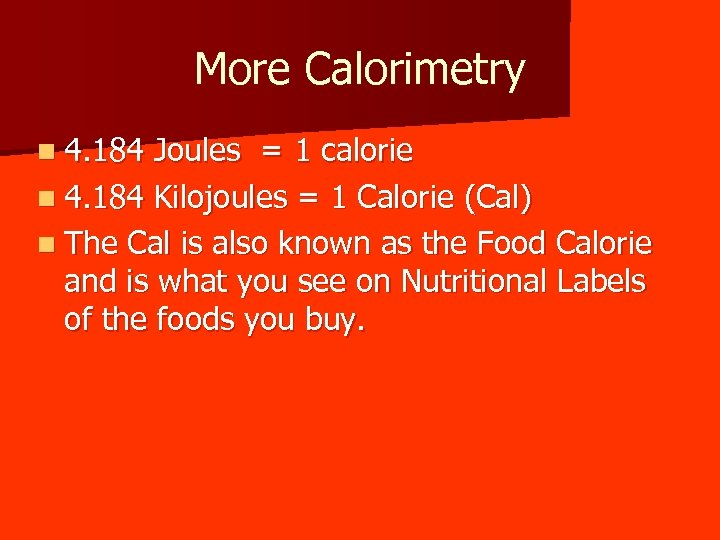More Calorimetry n 4. 184 Joules = 1 calorie n 4. 184 Kilojoules = 1 Calorie (Cal) n The Cal is also known as the Food Calorie and is what you see on Nutritional Labels of the foods you buy.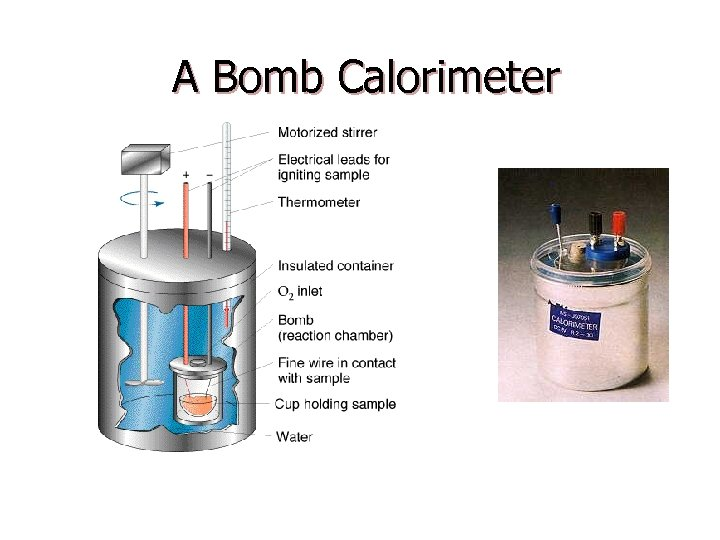A Bomb Calorimeter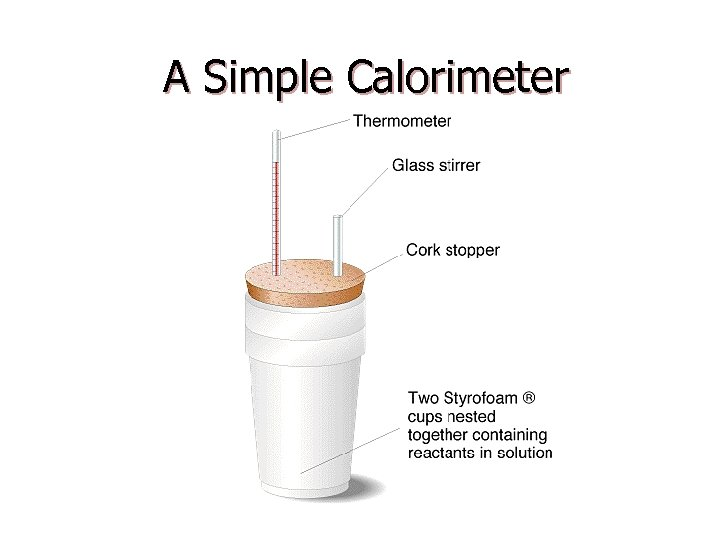A Simple Calorimeter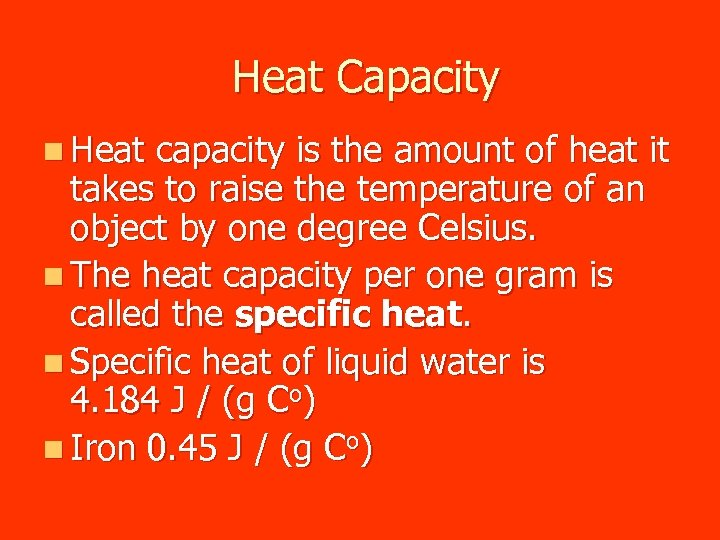Heat Capacity n Heat capacity is the amount of heat it takes to raise the temperature of an object by one degree Celsius. n The heat capacity per one gram is called the specific heat. n Specific heat of liquid water is 4. 184 J / (g Co) n Iron 0. 45 J / (g Co)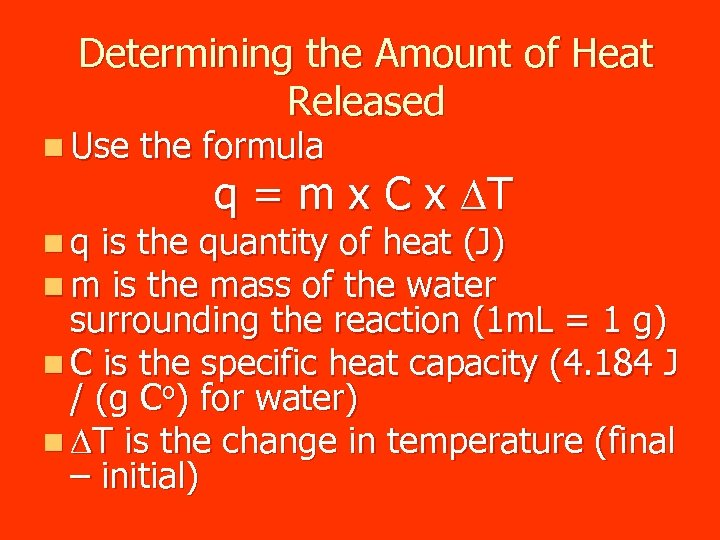Determining the Amount of Heat Released n Use the formula q = m x C x DT n q is the quantity of heat (J) n m is the mass of the water surrounding the reaction (1 m. L = 1 g) n C is the specific heat capacity (4. 184 J / (g Co) for water) n DT is the change in temperature (final – initial)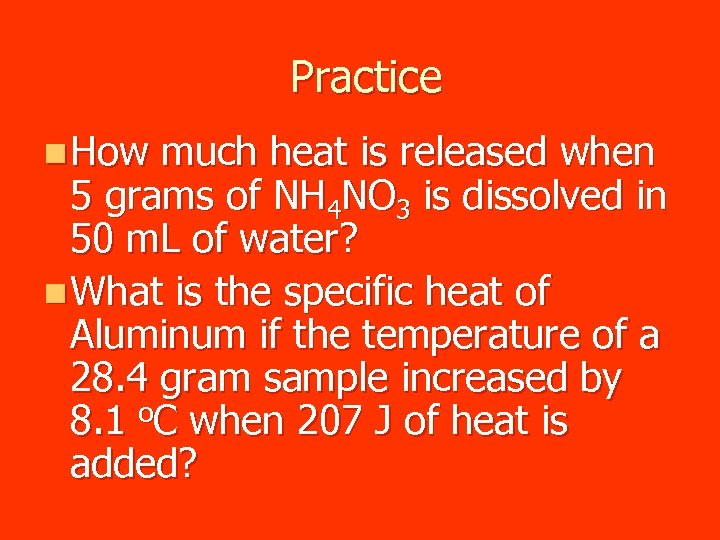Practice n How much heat is released when 5 grams of NH 4 NO 3 is dissolved in 50 m. L of water? n What is the specific heat of Aluminum if the temperature of a 28. 4 gram sample increased by 8. 1 o. C when 207 J of heat is added?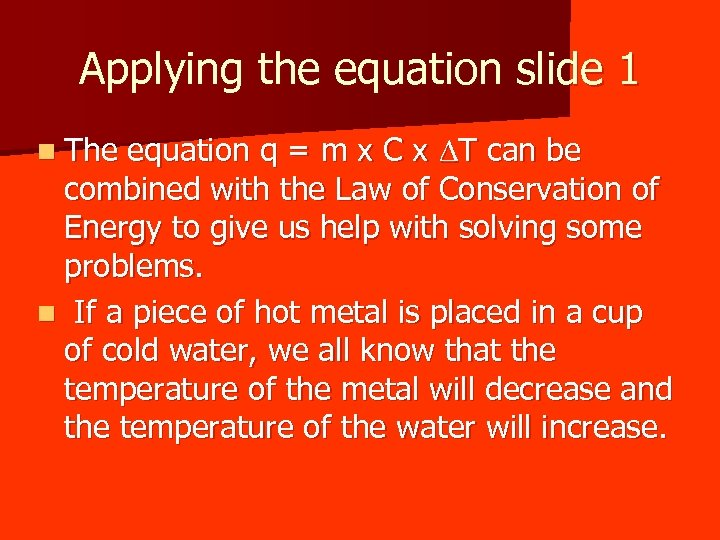Applying the equation slide 1 equation q = m x C x DT can be combined with the Law of Conservation of Energy to give us help with solving some problems. n If a piece of hot metal is placed in a cup of cold water, we all know that the temperature of the metal will decrease and the temperature of the water will increase. n The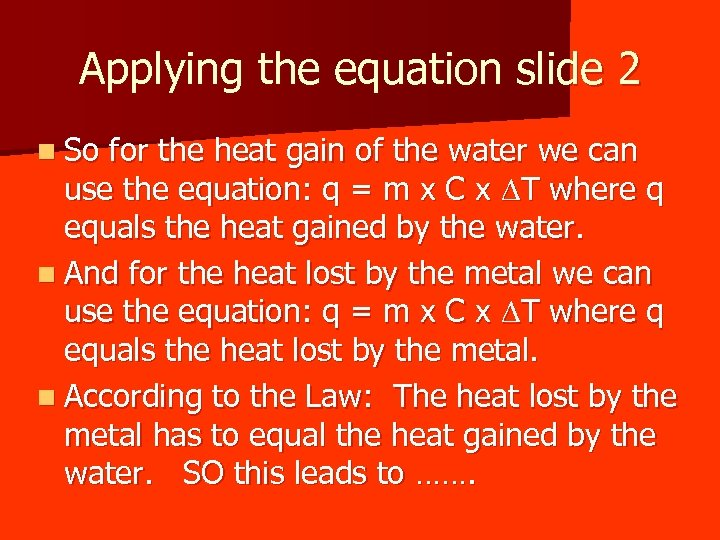Applying the equation slide 2 n So for the heat gain of the water we can use the equation: q = m x C x DT where q equals the heat gained by the water. n And for the heat lost by the metal we can use the equation: q = m x C x DT where q equals the heat lost by the metal. n According to the Law: The heat lost by the metal has to equal the heat gained by the water. SO this leads to …….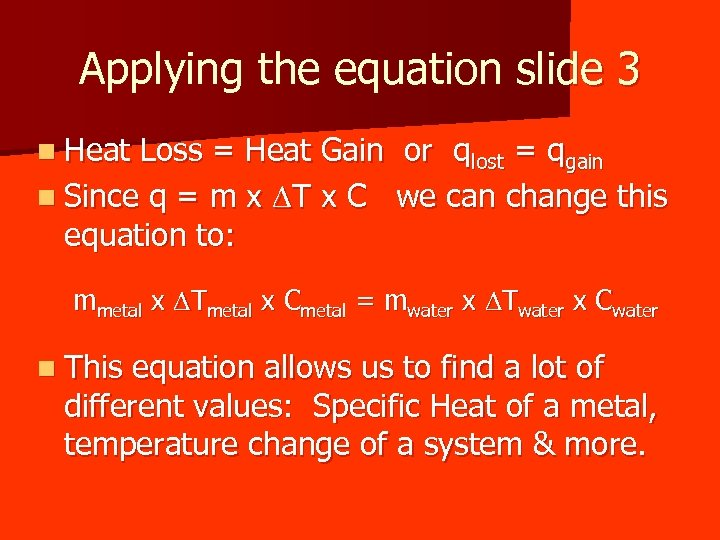Applying the equation slide 3 n Heat Loss = Heat Gain or qlost = qgain n Since q = m x DT x C we can change this equation to: mmetal x DTmetal x Cmetal = mwater x DTwater x Cwater n This equation allows us to find a lot of different values: Specific Heat of a metal, temperature change of a system & more.Working our new equation n Remember to watch which variables are being added. In other words, remember to keep all the data for the water together on the same side of the equation and do the same for the other material as well. n If you are having problems with the algebra, don’t forget to watch the solved problems done in class.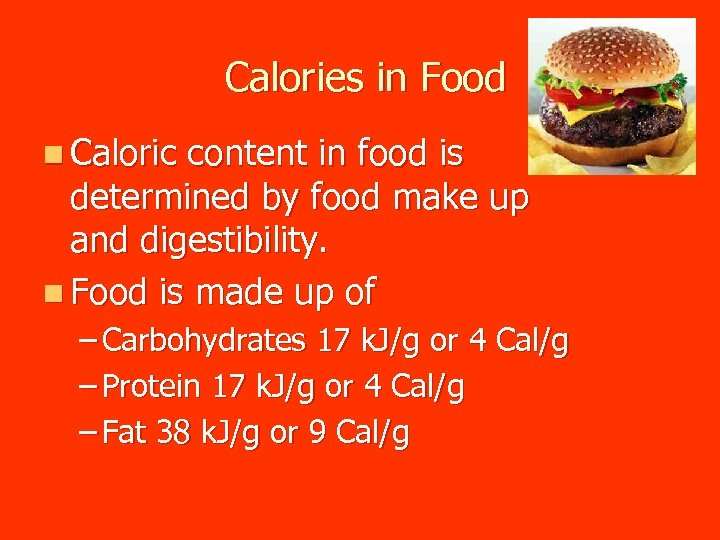Calories in Food n Caloric content in food is determined by food make up and digestibility. n Food is made up of – Carbohydrates 17 k. J/g or 4 Cal/g – Protein 17 k. J/g or 4 Cal/g – Fat 38 k. J/g or 9 Cal/g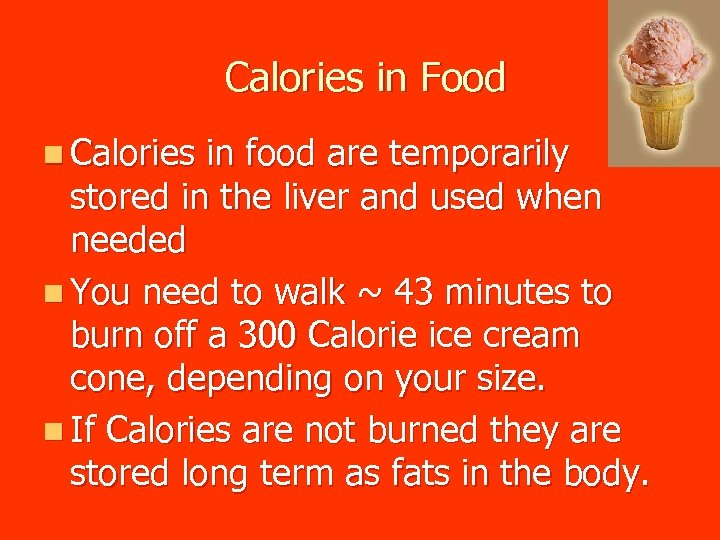Calories in Food n Calories in food are temporarily stored in the liver and used when needed n You need to walk ~ 43 minutes to burn off a 300 Calorie ice cream cone, depending on your size. n If Calories are not burned they are stored long term as fats in the body.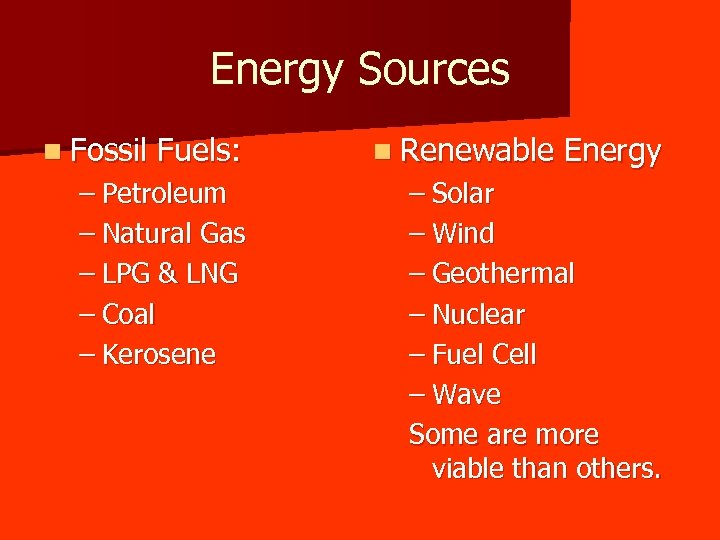Energy Sources n Fossil Fuels: – Petroleum – Natural Gas – LPG & LNG – Coal – Kerosene n Renewable Energy – Solar – Wind – Geothermal – Nuclear – Fuel Cell – Wave Some are more viable than others.